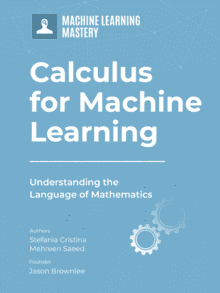# A Gentle Introduction to Derivatives of Powers and Polynomials

One of the most frequently used functions in machine learning and data science algorithms are polynomials or functions involving powers of x. It is therefore, important to understand how the derivatives of such functions are calculated.

In this tutorial, you will discover how to compute the derivative of powers of x and polynomials.

After completing this tutorial, you will know:

• General rule for computing the derivative of polynomials
• General rule for finding the derivative of a function that involves any non-zero real powers of x

Let’s get started.

## Tutorial Overview

This tutorial is divided into two parts; they are:

1. The derivative of a function that involve integer powers of x
2. Differentiation of a function that has any real non-zero power of x

## Derivative of the Sum of Two Functions

Let’s start by finding a simple rule that governs the sum of two functions. Suppose we have two functions f(x) and g(x), then the derivative of their sum can be found as follows. You can refer to the definition of the derivative, in case you need to review it.

Here we have a general rule that says that the derivative of the sum of two functions is the sum of the derivatives of the individual functions.

## Derivative of Integer Powers of x

Before we talk about derivatives of integer powers of x, let’s review the Binomial theorem, which tells us how to expand the following expression (here C(n,k) is the choose function):

(a+b)^n = a^n + C(n,1)a^(n-1)b + C(n,2)a^(n-2)b^2 + … + C(n,n-1)ab^(n-1) + b^n

We’ll derive a simple rule for finding the derivative of a function that involves x^n, where n is an integer and n>0. Let’s go back to the definition of a derivative discussed in this tutorial and apply it to kx^n, where k is a constant.

Following are some examples of applying this rule:

• Derivative of x^2 is 2x
• Derivative of 3x^5 is 15x^4
• Derivative of 4x^9 is 36x^8

## How to Differentiate a Polynomial?

The two rules, i.e., the rule for the derivative of the sum of two functions, and the rule for the derivative of an integer power of x, enable us to differentiating a polynomial. If we have a polynomial of degree n, we can consider it as a sum of individual functions that involve different powers of x. Suppose we have a polynomial P(x) of degree n, then its derivative is given by P'(x) as:

This shows that the derivative of the polynomial of degree n, is in fact a polynomial of degree (n-1).

## Examples

Some examples are shown below, where the polynomial function and its derivatives are all plotted together. The blue curve shows the function itself, while the red curve is the derivative of that function.

## What about Non-Integer Powers of x?

The rules derived above extend to non-integer real powers of x, which can be fractions, negative numbers or irrational numbers. The general rule is given below, where a and k can be any real numbers not equal to zero.

f(x) = kx^a

f'(x) = kax^(a-1)

A few examples are:

• Derivative of x^(0.2) is (0.2)x^(-0.8)
• Derivative of x^(𝜋) is 𝜋x^(𝜋-1)
• Derivative of x^(-3/4) is (-3/4)x^(-7/4)

Here are a few examples, which are plotted along with their derivatives. Again, the blue curve denotes the function itself, and the red curve denotes the corresponding derivative:

## Extensions

This section lists some ideas for extending the tutorial that you may wish to explore.

• Rules for derivatives of the product of two functions
• Rules for derivatives of rational functions
• Integration

If you explore any of these extensions, I’d love to know. Post your findings in the comments below.

This section provides more resources on the topic if you are looking to go deeper.

### Books

• Thomas’ Calculus, 14th edition, 2017. (based on the original works of George B. Thomas, revised by Joel Hass, Christopher Heil, Maurice Weir)
• Calculus, 3rd Edition, 2017. (Gilbert Strang)
• Calculus, 8th edition, 2015. (James Stewart)

## Summary

In this tutorial, you discovered how to differentiate a polynomial function and functions involving a sum of non-integer powers of x.

Specifically, you learned:

• Derivative of the sum of two functions
• Derivative of a constant multiplied by an integer power of x
• Derivative of a polynomial function
• Derivative of a sum of expressions involving non-integers powers of x

## Get a Handle on Calculus for Machine Learning!#### Feel Smarter with Calculus Concepts

...by getting a better sense on the calculus symbols and terms

Discover how in my new Ebook:
Calculus for Machine Learning

It provides self-study tutorials with full working code on:
differntiation, gradient, Lagrangian mutiplier approach, Jacobian matrix, and much more...